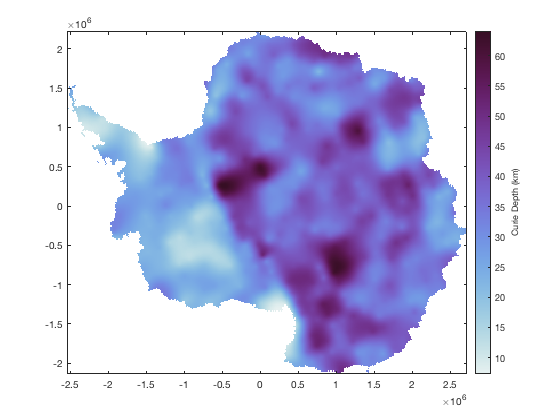# xyz2grid documentation

xyz2grid converts regularly-spaced columnated x,y,z data into gridded data. See also xyzread.

Back to Climate Data Tools Contents

## Syntax

```Z = xyz2grid(x,y,z)
Z = xyz2grid(filename)
Z = xyz2grid(filename,Name,Value)
[X,Y,Z] = xyz2grid(...)```

## Description

Z = xyz2grid(x,y,z) assumes x and y have some form of regularity and puts the corresponding values of z into a regular 2D MxN gridded matrix Z.

Z = xyz2grid(filename) loads data from a .xyz file of three columns (x, y, and z) then puts the data into a grid. This function assumes the input x,y,z data have some gridded regularity, but may have some missing data points.

Z = xyz2grid(filename,Name,Value) opens a .xyz file with any textscan options Name,Value, for example, 'headerlines',1.

[X,Y,Z] = xyz2grid(...) returns 2D meshgridded X and Y matrices corresponding to the values in Z.

## Example 1

You may have some columns of x,y, and z values that look like this:

```x = [1 1 2 2 2 3 3];
y = [1 2 1 2 3 1 3];
z = [1 2 4 5 6 7 9];

scatter(x,y,500,z,'filled')
axis([0 4 0 4])
colorbar
```From the scatter plot above, you can see that there is some gridded regularity to the data, even though a couple of spots in the grid are missing. That's okay. Let's grid it up:

```[X,Y,Z] = xyz2grid(x,y,z)
```
```X =
1     2     3
1     2     3
1     2     3
Y =
3     3     3
2     2     2
1     1     1
Z =
NaN     6     9
2     5   NaN
1     4     7
```

You can see the two missing values are filled by NaN. You can plot the gridded data with surf(X,Y,Z) or pcolor(X,Y,Z), but let's use imagescn instead:

```imagescn(X,Y,Z)
axis([0 4 0 4])
colorbar
```## Example 2: Antarctic Curie Depth

For this example, use load and plot Antarctic Curie depth data from Martos 2017:

```[X,Y,CD] = xyz2grid('Curie_Depth.xyz');

figure
imagescn(X,Y,CD)
cmocean dense

cb = colorbar;
ylabel(cb,'Curie Depth (km)')
```## Author Info

This function is part of the Climate Data Toolbox for Matlab. The function and supporting documentation were written by Chad A. Greene of the University of Texas at Austin.